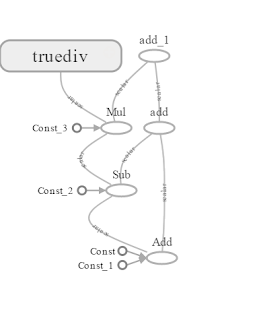## Posts

Showing posts from July, 2017

### Arithmetic Operations in TensorflowIn this article, we will learn about basic arithmetic operators supported by the Tensorflow. We will also perform these operations on the tensors and create a computational graph which can be viewed with the help of the Tensorboard. To get started, let's create two tensors of type constants. # Create two nodes node1 = tf.constant(35)  # dtype is int32 node2 = tf.constant(76)  # dtype is int32 You can add these two tensors using tf.add # Add node1 and node2 node_add = tf.add(node1, node2)  # dtype is int32 Ok now, lets learn how to subtract the two nodes. For this, I'm going to take our previous added result node_add as the first node and node3 as the second node. #  Create node3 node3 = tf.constant(11)  # dtype is int32 # Subtract node_add and node3 node_subtract = tf.subtract(node_add, node3)  # dtype is int32 Similarly, we use node_subtract, node4 for multiplication and node_multiply, node5 for division once we created the node4, node5. # Create node4 node4 =